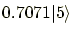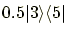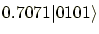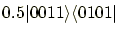Print the current state

1. Decimal representation
show (basis vector labels);
shows the component states (the complex amplitudes and the basis vectors) of specified labels in the style ofif the current state is kept as a vector. When the current state is represented as a density matrix, this command shows all the non-zero elements in the style of, ignoring the basis vector labels.
show;
shows all the non-zero elements of the current state in the decimal representation.
2. Binary representation
showb (basis vector labels);
shows the component states (the complex amplitudes and the basis vectors) of specified labels in the style ofif the current state is kept as a vector. When the current state is represented as a density matrix, this command shows all the non-zero elements in the style of, ignoring the basis vector labels.
showb;
shows all the non-zero elements of the current state in the binary representation.

root
2004-11-07